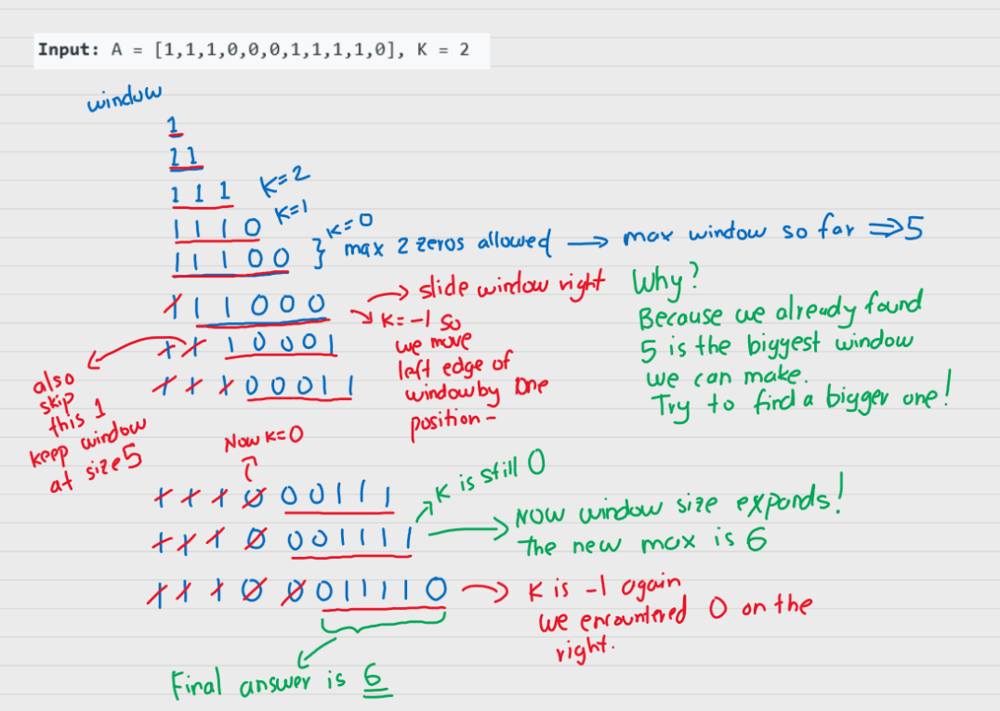# LC 1004 [M] Max Consecutive Ones III - codewithsenpai/algos Wiki

consecutive => sliding window

you can flip at most k 0's``````class Solution:
def longestOnes(self, A: List[int], K: int) -> int:
left = right = 0

for right in range(len(A)):
# if we encounter a 0 the we decrement K
if A[right] == 0:
K -= 1
# else no impact to K

# if K < 0 then we need to move the left part of the window forward
# to try and remove the extra 0's
if K < 0:
# if the left one was zero then we adjust K
if A[left] == 0:
K += 1
# regardless of whether we had a 1 or a 0 we can move left side by 1
# if we keep seeing 1's the window still keeps moving as-is
left += 1

return right - left + 1
``````
``````class Solution:
def longestOnes(self, A: List[int], k: int) -> int:
max_len = 0
consecutive = 0

l = 0
for r in range(len(A)):
if A[r] == 1:
consecutive += 1

if (r - l + 1) - consecutive > k:
if A[l] == 1:
consecutive -= 1
l += 1

max_len = max(max_len, r - l + 1)

return max_len
``````